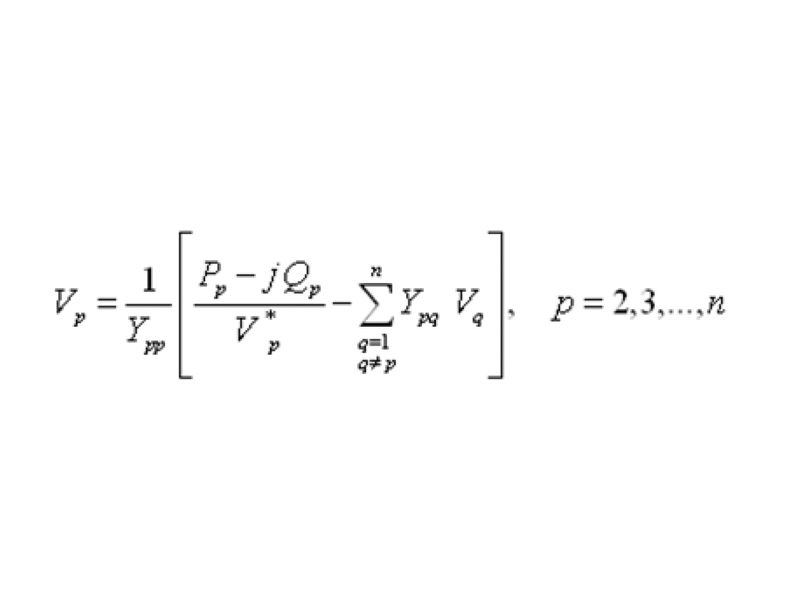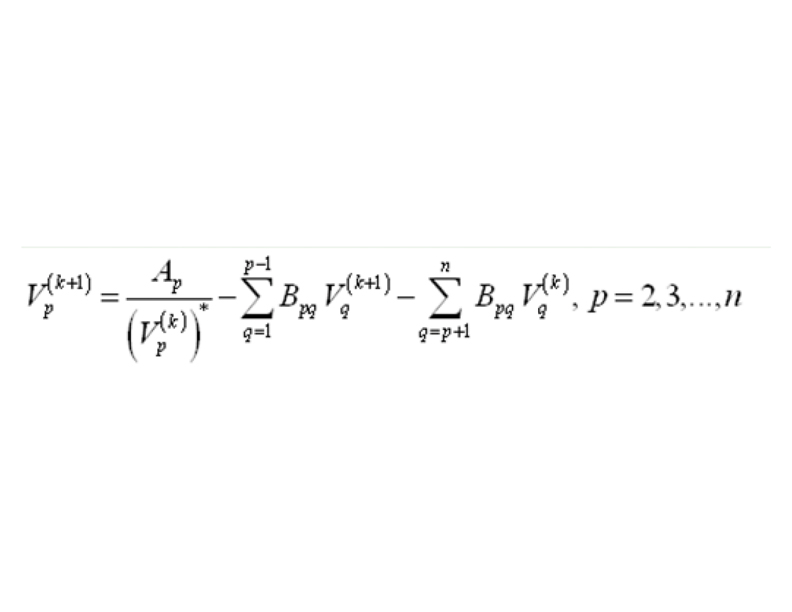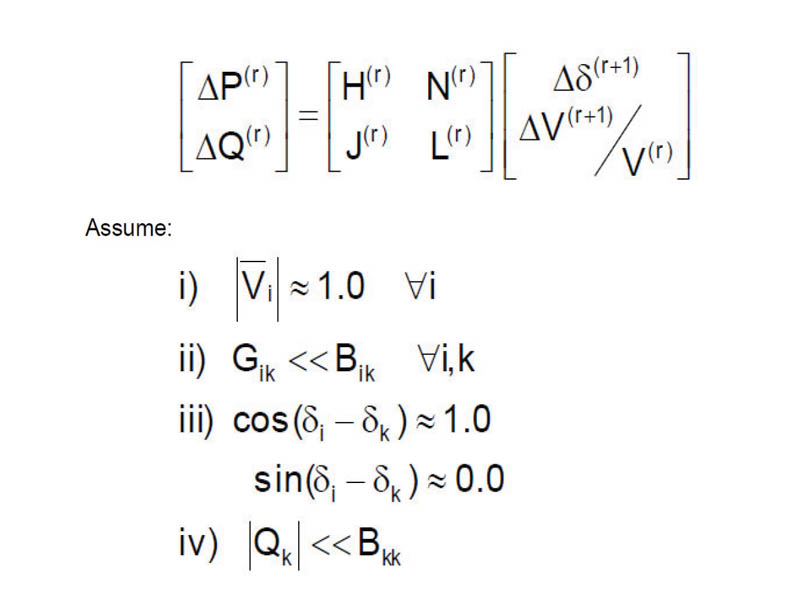# LOAD FLOW ANALYSIS THROUGH ITERATIVE TECHNIQUES

In this article, authors have discussed various Load Flow Analysis techniques such as Gauss-Seidel method, Newton-Raphson Method and Fast-decoupled method. With a schematic case study, they have explained a 3 bus power system study, and results of load flow studies are also presented. Power World Simulator is used for modeling the power system. Comparison and advantages, disadvantages of all methods are listed out for selection of iterative technique...The Load Flow Analysis is a very fundamental and important tool in interconnected power system analysis. Load Flow Analysis results play major role during the operational stages of any power system for its control and economic schedule, as well as during the expansion or design stages. The main purpose of any Load Flow Analysis is to compute precise steady-state voltages and voltage angles of all buses in the network, the reactive and real power flows into every overhead trasnmission line and power transformer (located at Sub-stations), under the assumption of known generation and load. The load flow solution also gives the initial conditions of the system when the transient beheviour of the system is to be studied. In actual practice, it will be required to carry out numerous power flow solutions under a variety of conditions.

To understand the the load flow analysis, the knowledge of various Bus such as Load bus, Voltage controlled bus (Generator bus), Slack bus and thier data is required for applying any iterative techniques such as Gauss-Seidel method, Newton- Raphson Method and Fast-decoupled method.

Gauss- iteravie Method

The load flow problem can be formulated as a set of nonliner algebraic equations, which can be solved by an iterative algorithm called Gauss method. In this method, non linear equations can be solved by guessing the initinal solution approximately. Then, this guessed solution is used in conjuction with the original equation to cmpute a new and more accurate estimate for the solution can be used. The second estimate is used to find the third estimate. The process is continued until the difference between successive estimate is extremely negilible. The main disadvantage of this method is that it requires an extremely large number of iterations to reach sufficiently close to the solution, but with the use of computers it may not be thought of as a drawback. Another drawback is that this method may not converge, if the solution does not exist or if the iteration process is divergent.

Gauss-Seidal Method

Gauss-Seidel (G-S) method is one of the simplest iterate method. It is a modification of Gauss-Iterative method. This modification will reduce the numbers of iterations. So, it is suitable for the power flow study of small power system. To understnad the method, we can take two cases such as When PV Buses are absent and another is When PV Buses are present. Following schematic system is used to understnad the method. The schmetic system is simulated in Power World Simulator and it can show the Power Flow also.

Schematic diagram of Load Flow Analysis and Solution

Power Flow through Gauss Seidel Method:

Case Information: Case voltages set to flat start values

Generator MVAR outputs brought inside their limits

Starting Solution using Gauss-Seidel

Number: 0 Max P: 400.000 at bus 2 Max Q: 250.000 at bus 2

Number: 1 Max P: 107.118 at bus 2 Max Q: 53.786 at bus 2

NR PowerFlow – Single iteration complete

Solution Finished in 0.001 Seconds

Simulation: Power Flow did not converge!

Mathematical Equation Based on GS Method:

• PV Buses are Absent
• PV Buses are Present1. First repeat the iteration for PQ buses, then continue the iteration for PV buses.

2. At the PV buses, P & V are specified and Q & δ are unknown to be determined.

3. Therefore, the values of Q & δ are to be updated in every iteration through bus equations.

• Small Computer memory requirement
• Simplicity of technique
• Less Computational time per iteration.

• Increase of number of iterations directly with the increased number of buses.
• Slow rate of convergence and thus, large number of iterations.
• Effect on convergence due to choice of slack bus.

NR method:

• Let us assume that an n -bus power system contains a total np number of P-Q buses while the number of P-V (generator) buses be ng such that n = np + ng + 1.
• Bus-1 is assumed to be the slack bus.

The Newton-Raphson procedure is as follows:

Step-1: Choose the initial values of the voltage magnitudes |V|(0) of all np load buses and n − 1 angles δ(0) of the voltages of all the buses except the slack bus.

Step-2: Use the estimated |V|(0) and δ(0) to find out a total n − 1 number of injected real power Pcalc(0) and equal number of real power mismatch ΔP(0).

Step-3: Use the estimated |V|(0) and δ(0) to estimate a total np number of injected reactive power Qcalc(0) and equal number of reactive power mismatch ΔQ(0) .

Step-3: Use the estimated |V|(0) and δ(0) to make the Jacobian matrix J(0) .

Step-4: Solve for δ(0) and Δ |V|(0) ÷ |V|(0).

Step-5 : Obtain the updates fromStep-6: Check if all the mismatches are below a small number. Terminate the process if yes. Otherwise go back to step-1 to start the next iteration.

Power Flow through NR Method:

Case Information: Case voltages set to flat start values

Generator MVAR outputs brought inside their limits

Starting Solution using Rectangular Newton-Raphson

Number: 0 Max P: 400.000 at bus  2 Max Q:  250.000 at bus 2

Number: 1 Max P: 16.120 at bus  3 Max Q: 39.945 at bus 2

NR PowerFlow – Single iteration complete

Solution Finished in 0.000 Seconds

Simulation: Power Flow did not Converge!

• This Method is faster, more reliable and results are more accurate.
• Generally it’s suitable for large systems.

• The programming logic is more complex than GS method.
• As the numbers of iterations are more the requirement of computer memory is higher than GS method.

Fast Decoupled Method:

• Do not build Jacobian at each iteration (J constant)
• Decoupling between P-δ and Q-V (not recommended in system highly loaded and/or with low voltage levels)Power Flow through Fast De Coupled Method:

Case Information: Case voltages set to flat start values

Generator MVAR outputs brought inside their limits

Starting Solution using Fast Decoupled Power Flow

Number: 1 Max P: 400.000 at bus 2 Max Q:  421.258 at bus  2

NR Power Flow – Single iteration complete

Solution Finished in 0.000 Seconds

Simulation: Power Flow did not converge!

• This Method is easier because of linearization of the matrix equations.
• The power system where X/R ration is higher it’s suitable over there.
• Memory requirement is less compared to NR Method.

• Require more number of Iterations than NR method.

Conclusion:

Load Flow Analysis is iterative process. Though there are different techniques that used to figure out the flows of power and bus voltage magnitude. As per the above discussion and simulation result, in view point of Authors, there are pros and cons of different methods, depending upon the requirement of system different methods GS, NR and FDLF will be the used for Load Flow calculation.Prof. Minesh K. Joshi,
Assistant Professor, Department of Electrical
Enggg, A D Patel Institute of Technology
(ADIT), A Constituent College of CVM
University, Vallabh Vidyanagar, GujaratProf. Paresh R. Modha,
Assistant Professor, Department of Electrical
Enggg, A D Patel Institute of Technology
(ADIT), A Constituent College of CVM
University, Vallabh Vidyanagar, Gujarat